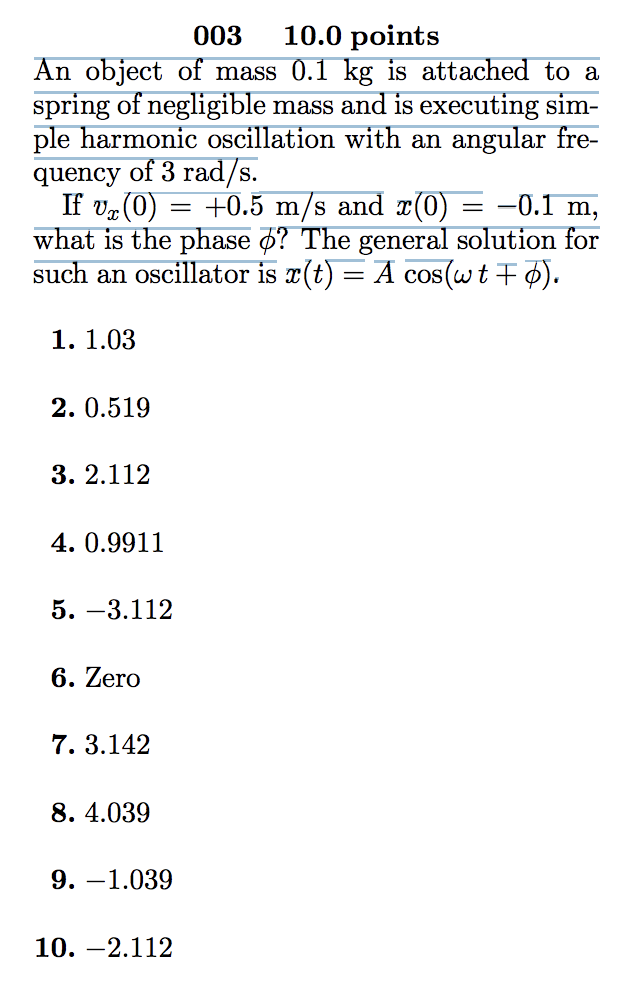# An object of mass 0.1 kg is attached to a spring of negligible mass and is...

###### Question:

An object of mass 0.1 kg is attached to a spring of negligible mass and is executing simple harmonic oscillation with an angular frequency of 3 rad/s. If vx(0) = +0.5 m/s and x(0) = −0.1 m, what is the phase φ? The general solution for such an oscillator is x(t) = A cos(ω t + φ).10.0 points mass 0.1 kg is attached to a mass and is executing sim- 003 An object of spring of negligible ple harmonic oscillation with an quency of 3 rad/s. If v (0) what is the phase ? The general solution for such an oscillator is r(t) = A cos(wt ) angular fre- +0.5 m/s and x(0) -0.1 m, = _ 1. 1.03 2. 0.519 3. 2.112 4.0.9911 5. -3.112 6. Zero 7.3.142 8. 4.039 9. -1.039 10. -2.112

#### Similar Solved Questions

##### How do you graph the equation by plotting points x – 2y = 6?
How do you graph the equation by plotting points x – 2y = 6?...